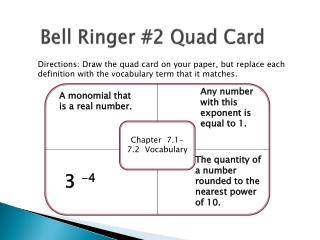DownloadDownload PresentationBell Ringer #2 Quad Card

# Bell Ringer #2 Quad Card

Download Presentation## Bell Ringer #2 Quad Card

- - - - - - - - - - - - - - - - - - - - - - - - - - - E N D - - - - - - - - - - - - - - - - - - - - - - - - - - -
##### Presentation Transcript

1. Bell Ringer #2 Quad Card Directions: Draw the quad card on your paper, but replace each definition with the vocabulary term that it matches. Any number with this exponent is equal to 1. A monomial that is a real number. Chapter 7.1-7.2 Vocabulary The quantity of a number rounded to the nearest power of 10. 3 -4

2. Chapter 7.2 NotesObjectives • SWBAT divide monomials using the properties of exponents. • SWBAT simplify expressions containing negative and zero exponents. • Students will reason abstractly and quantitatively.

3. Quotient of Powers

4. Example 1: • Simplify

5. Power of a Quotient

6. Example 2: Power of a Quotient

7. Zero Exponent Property

8. Example 3: Zero Exponent

9. Negative Exponent Property

10. Example 4: Negative Exponents

11. Example 5: Apply Properties of Exponents.

12. Quick Quiz Pg. 402 (2, 12, 14) HOMEWORK: pg. 403 (20-44 evens; 66-69 all)